output.to from Sideway
Number Theory

NumberPrime NumberFactorization

Draft for Information Only

# Content

``` Factorization   Prime Factorization Trial Division   Fermat Factorization   Pollard Rho Factorization ```

# Factorization

Factorization is one of the methods to verify whether a number is prime.

## Prime Factorization Trial Division

The direct search factorization is a bruce force factorization method for determining all possible prime factors of a composite number, N, through the repeated examination of divisibility by  trial division from a set of non-trivial prime divisors.

Let x, y are non-trivial factors of integer N with N=xy. imply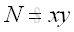It is not necessary to test all numbers from 1 to N-1.

If x, y are non-trivial factors of integer N with N=xy and x≤y, then x≤√N.

Since if x>√N, then y≥x>√N and imply xy>N, which contradicts to the assumption N=xy.

Therefore, the trial division can be performed by checking whether x divides N, x|N, for x=2 to the floor of √N. if x is found, imply x≡0 (mod N),  and y, y=N/x is a factor also.

In verifying whether a number N is prime,  x can be limited to a non-trivial prime factor.

## Fermat Factorization

The Fermat factorization (1600s) from Pierre de Fermat is another way of factoring a composite number by considering the composite number as the difference of squares.  This standard binomial can then be factorized into a product. Imply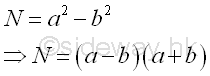Since all even number can be divided by 2, let x, y are non-trivial odd factors of integer N with N=xy. implyEquate two equations. Imply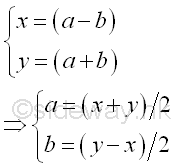Both a and b are integers because x and y are odd integers, the sum and difference between any two odd number are even number which is divisible by 2. As x and y are non trivial factors,  √N≥x>1 and y≥x, imply a≥1 and b≥0.

Instead of testing the non trivial factors x, and y, Fermat factorization examine the integers a and b. Imply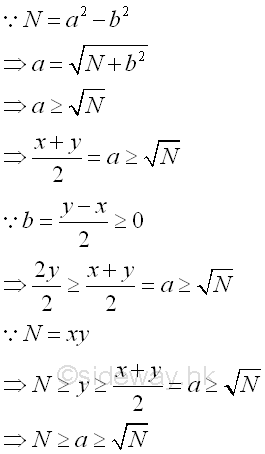Therefore, the Fermat factorization can be performed by checking a from the floor of √N to N. whether the corresponding value of b is an integer and the corresponding terms of the product, (a-b) and (a+y) are the non trivial factors of N. Imply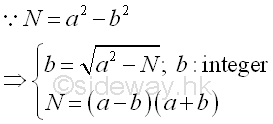In verifying whether a number N is prime,  the value of integer a should be choosen from the floor of √N to N.

## Pollard Rho Factorization

The Pollard rho factorization or Pollard's Monte Carlo factorization method (1975) from J.M. Pollard is another technique of finding factors of a composite number by making use of probabilistic ideas from transforming a sequencial sequence to a congruential pseudo-random sequence to increase the probability of getting prime factors of the composite number.

ID: 120400011 Last Updated: 17/4/2012 Revision: 0 Ref:References

1. R. Paulo, 1996, The New Book of Prime Number Records
2. Wolstenholme, R.J., 1862, On Certain Properties of Prime Numbers
3. Mann, H.B., Shanks D., 1972, A Necessary and Sufficient Condition for Primality, and Its Source
4. J.M Pollard, Kangaroos, 1975, A Monte Carlo Method for FactorizationHome 5

Management

HBR 3

Information

Recreation

Culture

Chinese 1097

English 337

Computer

Hardware 149

Software

Application 196

Numeric 19

Programming

Web 621

HTML 65

CSS 58

ASP.NET 62

OS 389

Knowledge

Mathematics

Algebra 21

Number Theory 206

Geometry 18

Calculus 67

Engineering

Mechanical

Rigid Bodies

Statics 92

Dynamics 37

Control

Physics

Electric 23